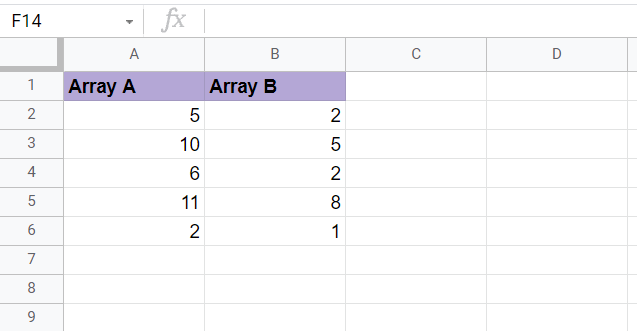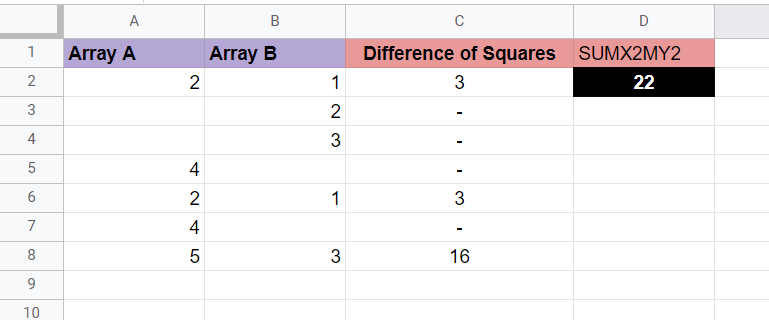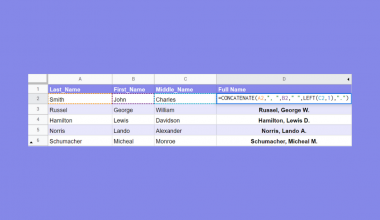# How to Use SUMX2MY2 Function in Google Sheets

The SUMX2MY2 function in Google Sheets is useful when you need to easily find the sum of the differences of the squares of values in two arrays.

This function is the Google Sheets function equivalent of calling  Σ(x²) where x and y correspond to values from two different arrays.

The rules for using the `SUMX2MY2` function in Google Sheets are as follows:

• The function requires two different arrays as input. They may also be cell ranges.
• The function outputs the sum of the differences of the squares of each pair of values in the two arrays

Let’s learn how to write the `SUMX2MY2` function ourselves in Google Sheets and later test out the function with actual values.

## The Anatomy of the SUMX2MY2 Function

So the syntax (the way we write) the DAYS function is as follows:

`=SUMX2MY2(array_x, array_y)`

Let’s dissect the function and understand what each of these terms means:

• = the equal sign is how we start any function in Google Sheets.
• SUMX2MY2() is our `SUMX2MY2` function. The function returns the sum of the difference of squares of corresponding values in the two provided arrays
• array_x refers to the array or cell range whose squares will be reduced by the squares of corresponding entries in array_y and later added together.
• array_y refers to the array or cell range whose squares will be subtracted from the squares of corresponding entries in array_x and later added together.
• Do note that the order of arguments is important since subtraction is involved.

## A Real Example of Using SUMX2MY2 Function

Let’s look into an example of the `SUMX2MY2` function being used in a Google Sheet spreadsheet.

In the worksheet below, we have two arrays we want to perform the operation with. Column C manually computes the difference of the squares of each corresponding value pair in the two arrays. Cell C7 sums up all these differences, giving us 64.In cell D2, our SUMX2MY2 function easily outputs the same result without having to use any other function!

You can make a copy of the spreadsheet above using the link I have attached below.

If you’re interested in trying out the SUMX2MY2 function in Google Sheets, let’s begin writing it ourselves!

## How to Use SUMX2MY2 Function in Google Sheets

1. First, we should locate the two arrays we’ll be running through our function.1. To begin using the `SUMX2MY2` function, select the cell we will first put our function’s output. In this example, we’ll output the result in cell C1
2. Next, we just simply type the equal sign ‘=‘ to begin the function, followed by ‘SUMX2MY2(‘. Do note not to confuse this function with the similarly named `SUMX2PY` and `SUMXMY2` functions.
3. As seen in the image below, a tooltip box appears with info on the `SUMX2MY2` function. We can click on the arrow on the top-right-hand corner of the box to minimize the pop-up if necessary.4. The next step is to select the two cell ranges we want to run through our function. We can easily drag the cell ranges to achieve the formula below.5. Afterward, press the Enter key to evaluate our formula. We should be able to now see the final result in cell C1.1. What happens if my two arrays have a different number of values?
The `SUMX2MY2` function requires that both arrays have the same number of values. If the number of values is unequal, then the `SUMX2MY2` function will return a ‘#N/A‘ error value
2. What would happen if my ranges are equal but some rows are empty or invalid?
As seen in the table below, the `SUMX2MY2` will still evaluate arrays with missing values, as long as the arrays are of equal length. Only the valid pairs will be considered when computing the result. In the example below, the function only added the results of rows 2, 6, and 8.That’s everything you need to know to use the `SUMX2MY2` function in Google Sheets easily. This step-by-step guide shows how easy it is to find the difference of squares of corresponding values in two arrays in Google Sheets.

You can now use the `SUMX2MY2` functions in Google Sheets together with the various other Google Sheets formulas available to create useful spreadsheets to help boost your productivity and workflow.

Stay notified of new guides for Google Sheets just like this by subscribing to our newsletter!Our goal this year is to create lots of rich, bite-sized tutorials for Google Sheets users like you. If you liked this one, you'll love what we are working on! Readers receive ✨ early access ✨ to new content.

##### You May Also Like## How to Use PRODUCT Function in Google Sheets

The PRODUCT function in Google Sheets is used to return the result of multiplying a series of numbers…## How to Pick a Random Name from a Long List in Google Sheets

You can easily create a random draw winner selection tool to pick a random name from a long…## Remove Duplicates from Comma-Delimited Strings in Google Sheets

In this article, you will learn how you can use a simple formula to remove duplicates from comma-delimited…## How to Compare Comma-Separated Values in Google Sheets

This article will show you different ways we can use Google Sheets formulas to compare cells with comma-separated…